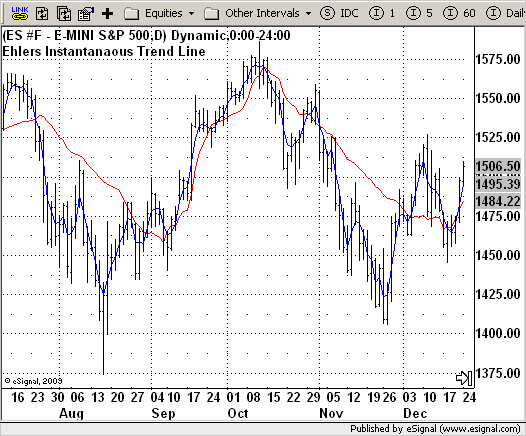# Instantaneous Trend Line (J. Ehler)

ICE Data Services -

File Name: ITL.efs

Description:
Instantaneous Trend Line (J. Ehler)

Formula Parameters:

Notes:

The early identification of a trend then depends on a valid measurement of short-term cyclic activity. There are two ways to do so, either by cycle elimination or by spectrum analysis. Of the two, cycle elimination is by far the easier.
The identification of the trend is that easy. One can eliminate the cyclic component when use the average over the cycle length.
One could adjust the average as the cycle length varies and plot the results day-by-day. The author called the result an "instantaneous trendline".

ITL.efsEFS Code:

```/*********************************
Provided By:
eSignal (Copyright c eSignal), a division of Interactive Data
Formula Script (EFS) is for educational purposes only and may be
modified and saved under a new file name.  eSignal is not responsible
for the functionality once modified.  eSignal reserves the right
to modify and overwrite this EFS file with each new release.

Description:
Instantaneous Trend Line (J. Ehler)

Version:            1.0  05/18/2009

Formula Parameters:                     Default:

Notes:
The early identification of a trend then depends on a valid
measurement of short-term cyclic activity. There are two ways
to do so, either by cycle elimination or by spectrum analysis.
Of the two, cycle elimination is by far the easier.
The identification of the trend is that easy. One can eliminate
the cyclic component when use the average over the cycle length.
One could adjust the average as the cycle length varies and plot
the results day-by-day. The author called the result an "instantaneous
trendline".
by John F. Ehlers article published in 10/1990 Stocks&Commodities magazine.
**********************************/
var bInit = false;

function preMain() {
setPriceStudy(true);
setStudyTitle("Ehlers Instantanaous Trend Line");
setCursorLabelName("TR",0);
setDefaultBarFgColor(Color.red,0);
setCursorLabelName("ZL",1);
setDefaultBarFgColor(Color.blue,1);
}

var xValue1 = null;
var xInPhase = null;
var xPhase = null;
var xDeltaPhase = null;
var xInstPeriod = null;
var xTrendline = null;

function main(){
var Trendline = 0;
var	value11 = 0;
if (bInit == false) {
xValue1 = mom(6);
xInPhase = ema(6, xValue1);
xDeltaPhase = efsInternal("Calc_DeltaPhase", xPhase);
xInstPeriod = ema(8, efsInternal("Calc_InstPeriod", xDeltaPhase));
xTrendline = efsInternal("Calc_Trendline", xInstPeriod);
xvalue11 = ema(6, efsInternal("Calc_value11"));
bInit = true;
}
Trendline = xTrendline.getValue(0);
value11 = xvalue11.getValue(0);
if (value11 == null || Trendline == null) return;
return new Array(Trendline, value11);
}

function Calc_Value3(xValue1) {
var nRes = 0;
nRes = 0.75 * (xValue1.getValue(0) - xValue1.getValue(-6)) + 0.25 * (xValue1.getValue(-2) - xValue1.getValue(-4));
return nRes;
}

var Phase = 0;
var	InPhase = xInPhase.getValue(0);
var	InPhase_1 = xInPhase.getValue(-1);
if(Math.abs(InPhase + InPhase_1) > 0)
if(InPhase < 0 && Quadrature > 0)
Phase = 180 - Phase;
if(InPhase < 0 && Quadrature < 0)
Phase = 180 + Phase;
if(InPhase > 0 && Quadrature < 0)
Phase = 360 - Phase;
return Phase;
}

function Calc_DeltaPhase(xPhase) {
var DeltaPhase = 0;
var Phase = xPhase.getValue(0);
var Phase_1 =  xPhase.getValue(-1);
DeltaPhase = Phase_1 - Phase;
if(Phase_1 < 90 && Phase > 270)
DeltaPhase = 360 + Phase_1 - Phase;
if(DeltaPhase < 1)
DeltaPhase = 1;
if(DeltaPhase > 60)
Deltaphase = 60;
return DeltaPhase;
}

function Calc_InstPeriod(xDeltaPhase) {
var	InstPeriod = 0;
var InstPeriod_1 = ref(-1);
var	value4 = 0;
var i = 0;
if (xDeltaPhase.getValue(-40) == null) return;
for(i = 0; i <= 40; i++){
value4 += xDeltaPhase.getValue(-i);
if(value4 > 360 && InstPeriod == 0)
InstPeriod = i;
}
if(InstPeriod == 0)
InstPeriod = InstPeriod_1;
return InstPeriod;
}

var xClose = null;

function Calc_Trendline(xInstPeriod) {
var value5 = xInstPeriod.getValue(0);
var tmp = 0;
var Period = 0;
var Trendline = 0;
if (xClose == null) xClose = close();
if (value5 == null) return;
if(value5 < 0){
tmp = - value5;
Period = - Math.floor(tmp);
} else
Period = Math.floor(value5);

Trendline = 0;
for(i = Period + 1; i >= 0; i--)
Trendline += xClose.getValue(-i);
if(Period > 0)
Trendline = Trendline / (Period + 2);
return Trendline;
}

var bSecondInit = false;
var xSecMom = null;

function Calc_value11() {
var value11 = 0;
if (bSecondInit == false) {
xSecMom = mom(3);
}
value11 = close(0) + 0.5 * xSecMom.getValue(0);
return value11;
}```